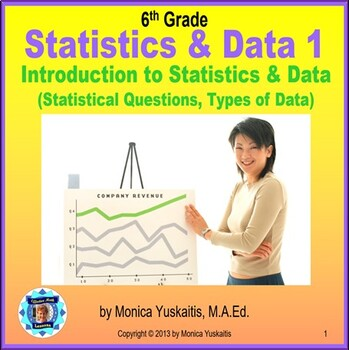# 6th Grade Statistics & Data 1 - Statistical Questions & Types of Data LessonSubject
Common Core Standards
Product Rating
4.0
6 Ratings
File Type

Presentation (Powerpoint) File

Be sure that you have an application to open this file type before downloading and/or purchasing.

4 MB|48 pages
Share
Also included in:
1. Sixth Grade Statistics and Data Bundle is composed of 6 different lessons comprising 361 slides on the following: Statistics and Data 1 - Statistical Questions and Types of Data, Statistics and Data 2 - Mean, Median, Mode & Range, Statistics and Data 3 - Dot Plot and Data Distribution, Statisti
\$19.50
\$15.60
Save \$3.90
Product Description

Sixth Grade Statistics 1 - Statistical Questions and Types of Data teaches students how to recognize and pose a statistical question. Students also learn the difference between numerical and categorical data. They also learn the 4 basic steps in presenting data: posing a statistical question, gathering the data, displaying the data, and answering the question they posed. There are 13 strategies of effective teaching based on research. For best results, students should have white boards and dry erase markers. Included in this powerpoint lesson are the following:

• title slide
• focus slide
• objectives
• Students will be able to identify a statistical question.
• Students will be able to distinguish between numerical and categorical data.
• Students will understand the process for producing statistical data.
• essential question
• What is a statistical question and what types of data are there?
• vocabulary
• concept development
• step-by-step demonstrations on how to identify a statistical question, how to distinguish between numerical and categorical data, and how to produce statistical data
• problem solving with word problem
• reward slide

Be sure to follow my store to be alerted of new products. The first 48 hours is always 50% off. CLICK HERE

Don't forget to leave feedback on this lesson to earn points for purchasing other TpT products.

You may be interested in the following related powerpoint lesson:

Link - Common Core 6th Statistics 2 - Mean, Median, Mode and Range

Link - Common Core 6th Statistics 3 - Dot Plot and Data Distribution

Link - Common Core 6th Statistics 4 - Histogram

Link - Common Core 6th Statistics 5 - Box and Whisker Plot

Link - Common Core 6th Statistics 6 - Mean Absolute Deviation

Total Pages
48 pages